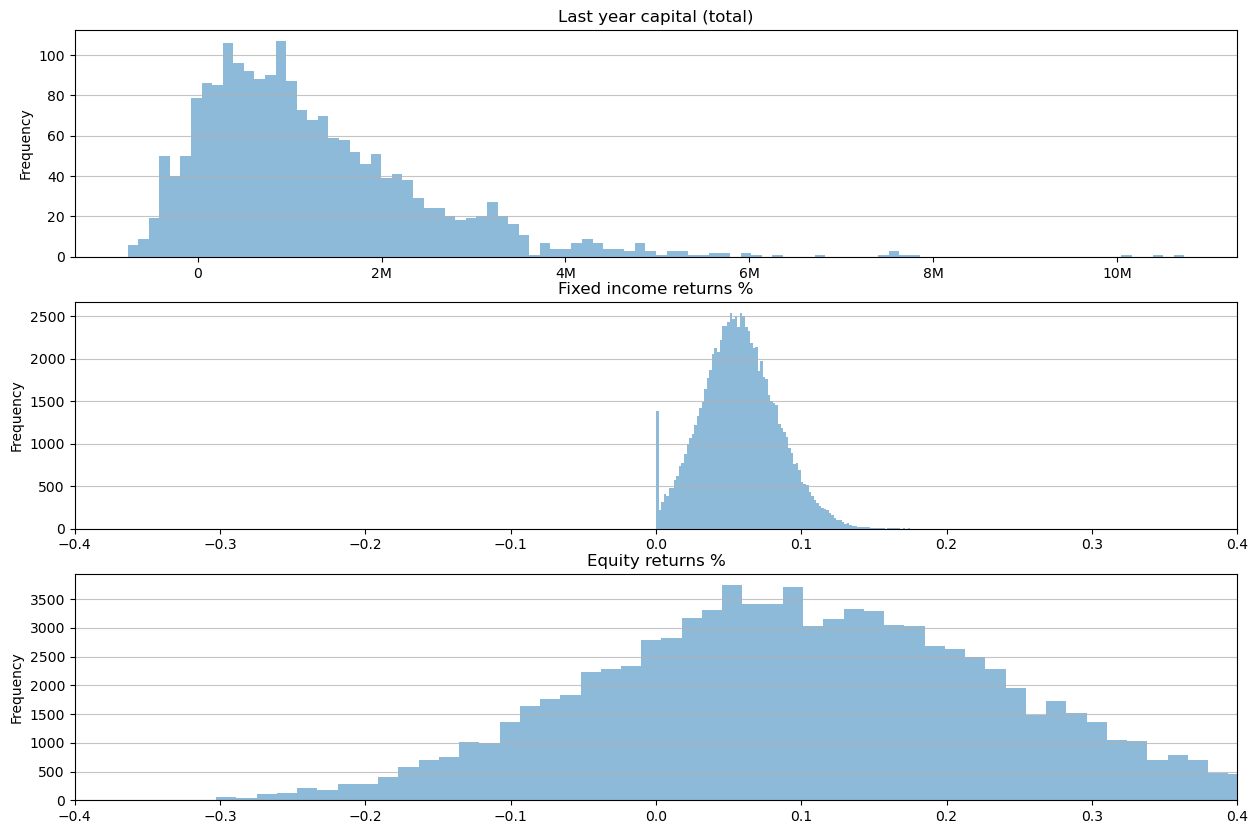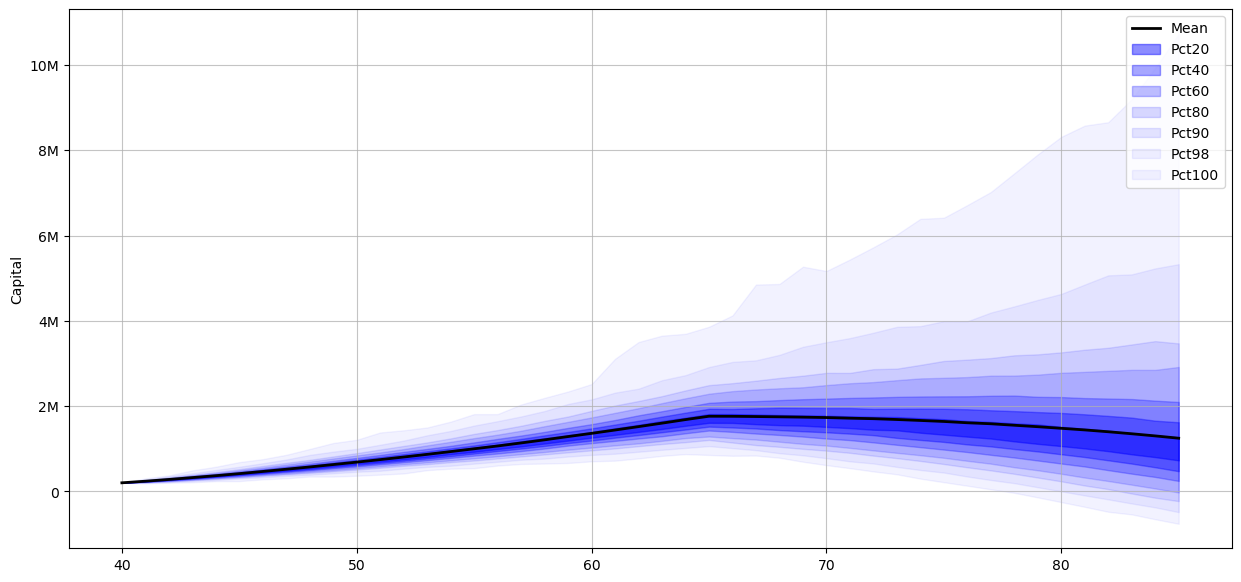# Notebook - Future Cash Flow Notebook⌗

This notebook performs basic personal income cash flow simulation. It uses probability distributions for interest rates, equity returns, and fixed income returns, and performs Monte Carlo methods to generate a histogram of possible cash flow outcomes.

The simulation takes salary, inflation, interest, tax, equity returns, rebalancing, and basic retirement income flow changes into account. There are US tax and Australian tax bracket simulations.

TODO:

• The probabilty distribution for the quantum harmonic oscillator to model returns (as per this paper) is slightly off. This notebook uses 1900+ S&P returns (including dividends) with a ‘fitted distribution’, but includes the QH model below (simply run that cell, instead of the S&P returns cell).
• best_fit_distribution should probably be replaced with fitter
• Properly check the math on interest rate modeling. Using mean reversion + jump diffusion, and looks similar to historical interest rate distribution, but should get another pair of eyes on it.

LIMITATIONS:

• No 401k/Superannuation tax modeling, nor pension income.
• Capital gains on equities is treated as income, which is clearly wrong. No application of long-term capital gains tax discounts.
• No simulation of primary residence/investment housing tax advantages, which are huge tax policy influences on capital outcomes.
• Fitting a distribution to the S&P is fraught with danger: a) the distribution can bias one way or another simply by resampling the distribution, b) S&P likely doesn’t give this distribution exposure to “dead companies” and is probably survivorship biased. One day I’ll grab all EOD price data for ASX and NYSE/NASDAQ, including dead companies and try and build a proper equities return model.
• Housing loans/repayments and returns aren’t modeled, and are a huge part of Australian investment.

Python 3.9.5: works on my machine[tm].

import sys
import os
import scipy.stats as st
import numpy as np
import pandas as pd
import matplotlib.pyplot as plt
import requests
import random
import warnings
import itertools

from matplotlib.ticker import FuncFormatter
from scipy.stats import norm, truncnorm, binom
from io import StringIO
# Google colab uses 3.8, so parser support for 'list' as type argument isn't there.
from typing import cast, Union, List, Tuple, Dict
from scipy.stats import truncnorm


### Helpers⌗

# helper fuctions
def human_format(num, pos):
magnitude = 0
while abs(num) >= 1000:
magnitude += 1
num /= 1000.0
# add more suffixes if you need them
return '%.f%s' % (num, ['', 'K', 'M', 'G', 'T', 'P'][magnitude])

formatter = FuncFormatter(human_format)

def flatten(l):
return [item for sublist in l for item in sublist]

def params_to_dict(params):
return {
'loc': params[-2],
'scale': params[-1],
'args': params[:-2],
}

def fit_distribution(data, distribution_function, bins=200):
# try and fit
y, x = np.histogram(data, bins=bins, density=True)
distribution = distribution_function
params = distribution.fit(data)

# Separate parts of parameters
arg = params[:-2]
loc = params[-2]
scale = params[-1]

# Calculate fitted PDF and error with fit in distribution
pdf = distribution.pdf(x, loc=loc, scale=scale, *arg)

return (x, pdf, params)

def best_fit_distribution(data, bins=200, ax=None):
"""Model data by finding best fit distribution to data"""
# Get histogram of original data
y, x = np.histogram(data, bins=bins, density=True)
x = (x + np.roll(x, -1))[:-1] / 2.0

# Distributions to check
DISTRIBUTIONS = [
st.alpha, st.anglit, st.arcsine, st.beta, st.betaprime, st.bradford, st.burr, st.cauchy, st.chi, st.chi2, st.cosine,
st.dgamma, st.dweibull, st.erlang, st.expon, st.exponnorm, st.exponweib, st.exponpow, st.f, st.fatiguelife, st.fisk,
st.foldcauchy, st.foldnorm, st.genlogistic, st.genpareto, st.gennorm, st.genexpon,
st.genextreme, st.gausshyper, st.gamma, st.gengamma, st.genhalflogistic, st.gilbrat, st.gompertz, st.gumbel_r,
st.gumbel_l, st.halfcauchy, st.halflogistic, st.halfnorm, st.halfgennorm, st.hypsecant, st.invgamma, st.invgauss,
st.invweibull, st.johnsonsb, st.johnsonsu, st.ksone, st.kstwobign, st.laplace, st.levy, st.levy_l,
st.logistic, st.loggamma, st.loglaplace, st.lognorm, st.lomax, st.maxwell, st.mielke, st.nakagami,
st.norm, st.pareto, st.pearson3, st.powerlaw, st.powerlognorm, st.powernorm, st.rdist, st.reciprocal,
st.rayleigh, st.rice, st.recipinvgauss, st.semicircular, st.t, st.triang, st.truncexpon, st.truncnorm, st.tukeylambda,
st.uniform, st.vonmises, st.vonmises_line, st.wald, st.weibull_min, st.weibull_max, st.wrapcauchy
]

# Best holders
best_distribution = st.norm
best_params = (0.0, 1.0)
best_sse = np.inf

# Estimate distribution parameters from data
for distribution in DISTRIBUTIONS:
# logging.info('fitting {}'.format(distribution))
# Try to fit the distribution
try:
# Ignore warnings from data that can't be fit
with warnings.catch_warnings():
warnings.filterwarnings('ignore')

# fit dist to data
params = distribution.fit(data)

# Separate parts of parameters
arg = params[:-2]
loc = params[-2]
scale = params[-1]

# Calculate fitted PDF and error with fit in distribution
pdf = distribution.pdf(x, loc=loc, scale=scale, *arg)
sse = np.sum(np.power(y - pdf, 2.0))

# if axis pass in add to plot
try:
if ax:
pd.Series(pdf, x).plot(ax=ax)  # type: ignore
except Exception:
pass

# identify if this distribution is better
if best_sse > sse > 0:
best_distribution = distribution
best_params = params
best_sse = sse

except Exception:
pass

return (best_distribution, best_params)

def get_truncated_normal(mean=0.0, sd=1.0, low=0.0, upp=10.0):
return truncnorm(
(low - mean) / sd, (upp - mean) / sd, loc=mean, scale=sd)

def create_fanchart(arr, figsize=(15, 10)):
x = np.arange(arr.shape)
# for the median use np.median and change the legend below
mean = np.mean(arr, axis=1)
offsets = (10, 20, 30, 40, 45, 49, 50)
fig, ax = plt.subplots(figsize=figsize)
ax.plot(mean, color='black', lw=2)
for offset in offsets:
low = np.percentile(arr, 50-offset, axis=1)
high = np.percentile(arr, 50+offset, axis=1)
# since offset will never be bigger than 50, do 55-offset so that
# even for the whole range of the graph the fanchart is visible
alpha = (55 - offset) / 100
ax.fill_between(x, low, high, color='blue', alpha=alpha)
ax.legend(['Mean'] + [f'Pct{2*o}' for o in offsets])
return fig, ax

from enum import Enum

class TaxRate(Enum):
Australia = 1,
UnitedStates = 2

# we're not doing tax credit holdover here, just a basic application
def apply_tax_year(
long_term_cap_flows: List[int],
short_term_cap_flows: List[int],
income_flows: List[int],
tax_rate: TaxRate = TaxRate.UnitedStates,
) -> int:
au_tax_brackets: list[int] = [
18201,
45001,
120001,
180001,
sys.maxsize,
]

au_tax_rates: list[float] = [
0.0,
0.19,
0.325,
0.37,
0.45
]

tax_brackets: list[int] = [
20550,
83551,
178151,
340101,
431901,
647851,
sys.maxsize,
]

tax_rates: list[float] = [
0.10,
0.12,
0.22,
0.24,
0.32,
0.35,
0.37,
]

if tax_rate == TaxRate.Australia:
tax_brackets = au_tax_brackets
tax_rates = au_tax_rates

# do this properly for US taxes
capital_gain_discount = 0.5
taxes = []

long_term_cap = sum(long_term_cap_flows) * capital_gain_discount

# apply
accumulated_flow = long_term_cap + sum(short_term_cap_flows) + sum(income_flows)
taxed_flow = 0.0

for index, bracket in enumerate(tax_brackets):
if accumulated_flow > bracket and index == len(tax_brackets) - 1:
tax_applied = (accumulated_flow - taxed_flow) * tax_rates[index]
taxes.append((tax_applied, bracket, taxed_flow, tax_rates[index]))
taxed_flow = accumulated_flow
break
elif accumulated_flow >= bracket:
tax_applied = (bracket - taxed_flow) * tax_rates[index]
taxes.append((tax_applied, bracket, taxed_flow, tax_rates[index]))
taxed_flow = bracket
elif accumulated_flow < bracket and taxed_flow < accumulated_flow:
tax_applied = (accumulated_flow - taxed_flow) * tax_rates[index]
taxes.append((tax_applied, bracket, taxed_flow, tax_rates[index]))
taxed_flow = accumulated_flow

tax_paid = sum([x for (x, _, _, _) in taxes])
return int(round(tax_paid))


## Set up the probability distributions for interest rates, equity returns and fixed income⌗

We use:

• Either: 1) fitted distribution to S&P returns data (including dividends) from 1920 onwards, or 2) Quantum harmonic oscillator for equity returns, modeled from this paper: https://iopscience.iop.org/article/10.1209/0295-5075/120/38003. (2) isn’t quite calibrated properly, so use caution.
• Historical interest rates (US Treasury 10 year, 1920 onwards) from the Federal Reserve, fitting a distribution over the histogram.
• Historical inflation data, (US CPI data, 1913 onwards). This is harder to ‘fit’ a distribution to, so we just sample from that historical data.
# get a distribution of interest rates stats
# this distribution is the current inflation rate for the given month. It doesn't need to be adjusted.
interest_rates_data = requests.get('https://datahub.io/core/bond-yields-us-10y/r/monthly.csv').text

ir_data = pd_interest_rates['Rate'] / 100
distribution, params = best_fit_distribution(ir_data, 30)

args = params_to_dict(params)
interest_rate_distribution = distribution(*args['args'], scale=args['scale'], loc=args['loc'])
interest_rate_distribution_array = interest_rate_distribution.rvs(15000)

fig, ax = plt.subplots()
_ = ax.hist(interest_rate_distribution_array, bins=100, density=True)
plt.xlim([-0.05, 0.25])
plt.show()

pd.DataFrame(interest_rate_distribution_array).describe()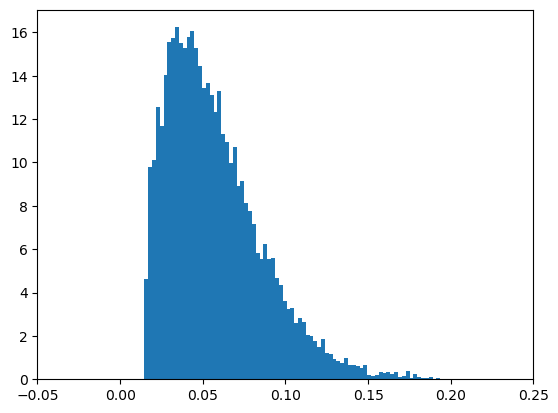.dataframe tbody tr th {
vertical-align: top;
}

text-align: right;
}


current_interest_rate = 0.0487
floor_rates = True

# interest rates modeled via mean reversion and jump diffusion using the Ornstein-Uhlenbeck process
def generate_interest_rates(T, start, mean, dt, mu, sigma, lambda_, eta, floor = False):
# T (float): Time horizon for the simulation.
# dt (float): Time step for the simulation.
# mu (float): Mean reversion rate. For example, a mu value of 0.05 means that the interest rate is expected to revert to its long-term mean of 0.05 at a rate of 0.05 per year.
# sigma (float): Volatility. mean +/- sigma
# lambda_ (float): Jump intensity. Expressed as the percentage chance of a jump in a given year (0.1 == 10%)
# eta (float): Mean jump size. For example, a eta value of 0.01 means that the interest rate is expected to jump by 0.01 standard deviations when a jump occurs. If the interest rate has a volatility of 0.05, then the expected size of a jump would be 0.01 * 0.05 = 0.0005.
N = int(T/dt)
interest_rates = np.zeros(N)
interest_rates = start

for i in range(1, N):
dW = np.random.normal(0, np.sqrt(dt))
jump = np.random.poisson(lambda_ * dt) * eta
interest_rates[i] = interest_rates[i-1] + mu * (start - interest_rates[i-1]) * dt + sigma * dW + jump

if floor:
interest_rates[interest_rates <= 0] = 0.0001
return interest_rates

# plot an example distribution
T = 30
# dt = 0.083 # 1/12 months
dt = 0.08

mu = 0.06
sigma = 0.0098
lambda_ = 0.1
eta = 0.008

plt.xlabel("Time (years)")
plt.ylabel("Interest Rate")
thirty_year_rates = []
last_year_rates = []
sample_rates = []

for i in range(0, 5000):
# sample from the historical distribution to get a mean
mean = random.choice(interest_rate_distribution_array)

# use the mean of the historical distribution as the mean for the interest rate
# mean = 0.057
interest_rates = generate_interest_rates(T, current_interest_rate, mean, dt, mu, sigma, lambda_, eta, floor_rates)
last_year_rates.append(interest_rates[-1])
thirty_year_rates.append(interest_rates)
if i % 20 == 0:
sample_rates.append(interest_rates)

for r in sample_rates:
plt.plot(np.arange(0, T, dt), r)

plt.show()

_ = plt.hist(last_year_rates, bins=100)
pd.DataFrame(last_year_rates).describe()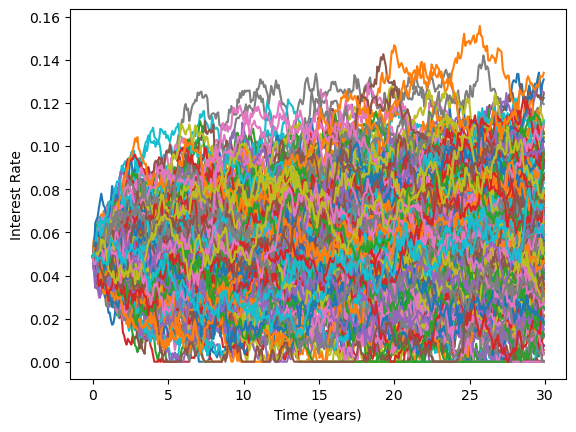.dataframe tbody tr th {
vertical-align: top;
}

text-align: right;
}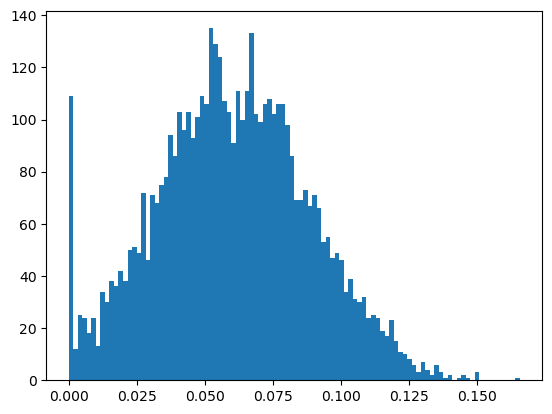# this model/distribution isn't calibrated (it skews far too negative), but I left it here for folks who want to tinker.
import numpy.polynomial.hermite as herm
import math

def qho_fp(x_range, t, C, mw, h_bar=1, n=5):
# This is taken from original paper, "Modeling stock return distributions with a quantum harmonic oscillator"
# Appears that t is the length of increments
pdf = []
for i in range(len(x_range)):
x = x_range[i]
p = 0
for i in range(n):
# En = n*h_bar*w
# p += (An / np.sqrt((s**n)*np.factorial(n))) * np.sqrt((m*w)/(pi*h_bar))*np.exp(-En*t) * \
# hermval(np.sqrt(m*w/h_bar)*x) * np.exp(-m*w*x**2/h_bar)
# Using the simplified fit formula from the paper
p_prime = C[i] * herm.hermval((mw / h_bar)**0.5 * x, i) * np.exp(-mw*x**2/(h_bar))
p += p_prime.real

if math.isinf(p) or math.isnan(p):
return pdf[0:i]
else:
pdf.append(p)

return pdf

def fit_qho(X0, parameters, t, data, N):
min_val = min(data)
max_val = max(data)
x_range = np.linspace(min_val, max_val, num=200)
qho = qho_fp(x_range, t, parameters[0:5], parameters)
pdf = [1000 * i for i in qho]
if len(qho) < len(x_range):
return None

else:
model = [X0]
sampling_vector = []
for i in range(len(pdf)):
for j in range(int(pdf[i])):
sampling_vector.append(x_range[i])

for i in range(len(data)):
model.append(np.random.choice(sampling_vector))

return model

# currently deprecated in favor of the cell below.
# model stock market returns. We're using Quantum Harmonic oscillator here. Code in quantum_harmonic.py
parameters = [0.2, 0.2, 0.086, 0.182, 0.133, 0.928]

harmonic_data = requests.get('https://zd8d32753f2xm110ee.s3.us-west-2.amazonaws.com/quantumharmonic.csv').text

daily_equity_qh_distribution_array = np.array(fit_qho(pd_harmonic_data, parameters, 1, pd_harmonic_data, 100)) / 100.0  # type: ignore

qh_equity_distribution_array = []
for i in range(30000):
start = 100
cumulative = 100
# 250 trading days in a year
for j in range(250):
r = random.choice(daily_equity_qh_distribution_array)
cumulative = cumulative + (cumulative * r)
qh_equity_distribution_array.append((cumulative - start) / start)

_ = plt.hist(qh_equity_distribution_array, bins=50)

pd.DataFrame(qh_equity_distribution_array).describe()

.dataframe tbody tr th {
vertical-align: top;
}

text-align: right;
}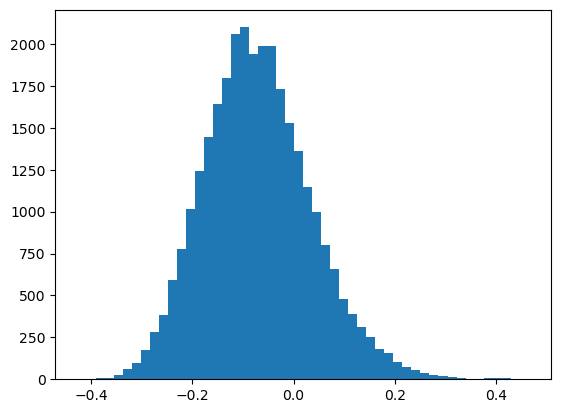# S&P 500 returns from 1900-2022
# https://www.officialdata.org/us/stocks/s-p-500/1900

sandp_data = requests.get('https://zd8d32753f2xm110ee.s3.us-west-2.amazonaws.com/sandp1900-2022.csv').text

distribution, params = best_fit_distribution(monthly_returns)
args = params_to_dict(params)

equity_distribution = distribution(*args['args'], scale=args['scale'], loc=args['loc'])
equity_distribution_rvs = equity_distribution.rvs(15000)

equity_distribution_array = []
for i in range(15000):
start = 100
cumulative = 100
for j in range(12):
r = random.choice(equity_distribution_rvs)
cumulative = cumulative + (cumulative * r)
equity_distribution_array.append((cumulative - start) / start)

_ = plt.hist(equity_distribution_array, bins=50, density=True)

pd.DataFrame(equity_distribution_array).describe()

.dataframe tbody tr th {
vertical-align: top;
}

text-align: right;
}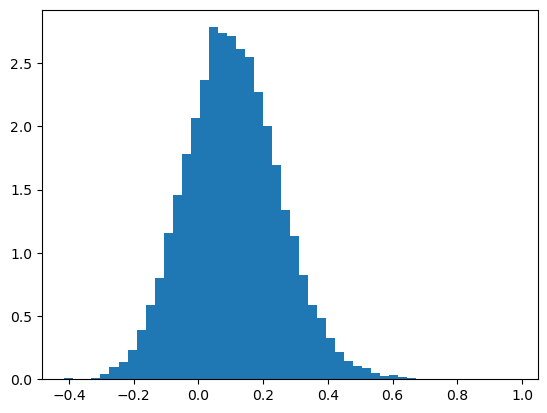# inflation data grabbed from the fed originally, and just dropped in as a list
inflation_data = np.array([0.02,0.09,0.18,0.13,0.1,0.16,-0.12,-0.08,0,-0.02,0.01,0.01,-0.01,0.01,0.01,-0.01,-0.1,-0.09,-0.04,0.01,0,0.02,0.04,0.01,-0.01,0.04,0.06,0.05,0.02,0.01,0.01,0.03,0.09,0.14,0.03,0.03,0.11,0.03,-0.01,0.01,0,0.01,0.03,0.02,0.01,0.01,0.01,0.01,0.02,0.02,0.02,0.04,0.04,0.04,0.05,0.03,0.03,0.05,0.07,0.11,0.11,0.08,0.08,0.09,0.09,0.1,0.12,0.11,0.06,0.04,0.04,0.04,0.04,0.04,0.05,0.05,0.06,0.01,0.02,0,0.02,0.02,0.02,0.01,0.02,0.03,0.03,0.02,0.03,0.02,0.02,0.02,0.02,0.02,0,0.02,0.03,0.02,0.01,0.02,0.01,0.01,0.02,0.02,0.02,0.01,0.03,0.07])
distribution, params = best_fit_distribution(inflation_data, bins=25)

args = params_to_dict(params)
inflation_rate_distribution = distribution(*args['args'], scale=args['scale'], loc=args['loc'])
inflation_rate_array = inflation_rate_distribution.rvs(15000)

fig, ax = plt.subplots()
_ = ax.hist(inflation_rate_array, bins=200)
plt.xlim([-0.25, 0.25])
plt.show()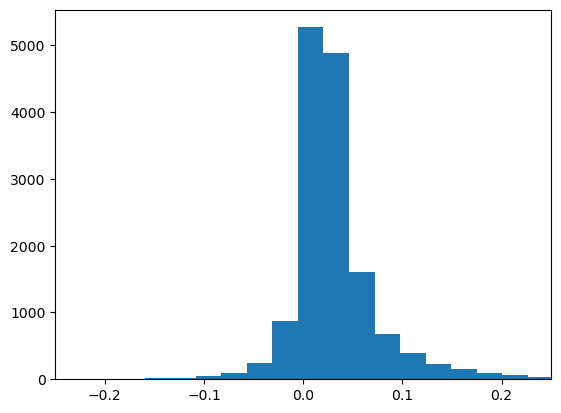## Code to simulate cash flow returns over time⌗

Call “sample”, with options:

• Current age, years remaining.
• Starting fixed income (cash, CD’s/term deposits)
• Starting equity (sum up your current equity holdings)
• Adjust for a basic stock/fixed rebalancing according to age.
• Apply US tax brackets (bool, False to apply Australian tax brackets)
# generate a dictionary where the keys are a persons age, and the value is the bond to equity ratio appropriate for that age
equity_fixed_ratio_by_age = {
value: 1 - (1 / (1 + np.exp(-0.1 * (value - 50)))) for (key, value) in enumerate(range(20, 90))
}

def sample_year_return(fixed: float, equity: float) -> Tuple[Tuple[float, float], Tuple[int, int]]:
fixed_return: float = random.choice(interest_rate_distribution_array)
equity_return: float = random.choice(equity_distribution_array)

fixed_post: float = fixed * fixed_return if fixed > 0 else 0
equity_post: float = equity * equity_return if equity > 0 else 0

return ((fixed_return, equity_return), (int(fixed_post), int(equity_post)))

def sample_year_return_interest_rate_simulation(
interest_rate_by_year: List[float],
year: int,
fixed: float,
equity: float
) -> Tuple[Tuple[float, float], Tuple[int, int]]:
fixed_return: float = interest_rate_by_year[year]
equity_return: float = random.choice(equity_distribution_array)

fixed_post: float = fixed * fixed_return if fixed > 0 else 0
equity_post: float = equity * equity_return if equity > 0 else 0

return ((fixed_return, equity_return), (int(fixed_post), int(equity_post)))

def sample_inflation() -> float:
return random.choice(inflation_data)

def rebalance_portfolio(fixed_to_equity_ratio: float, fixed: float, equity: float) -> Tuple[int, int]:
total = fixed + equity
fixed = total * (1 - fixed_to_equity_ratio)
equity = total * (fixed_to_equity_ratio)
return (int(fixed), int(equity))

def sample(
samples: int,
age: int,
retirement_age: int,
years_remaining: int,
starting_salary: int,
starting_expenses: int,
retirement_expenses: int,
starting_fixed_income: int,
starting_equity: int,
tax: bool = False,
tax_rate: TaxRate = TaxRate.UnitedStates,
rebalance: bool = False,
simulated_interest_rates: bool = False,
) -> List[Dict[str, List[Union[int, float]]]]:
current_interest_rate = 0.0487

return_samples: List[dict[str, List[Union[int, float]]]] = []

if simulated_interest_rates:
generated_interest_rates = []
for i in range(0, samples * 2):
monthly_rates = generate_interest_rates(
years_remaining + 1,
current_interest_rate,
0.058,
dt = 0.08,
mu = 0.06,
sigma = 0.0098,
lambda_ = 0.1,
eta=0.008,
floor=True
)
yearly_rates = monthly_rates[::12]
generated_interest_rates.append(yearly_rates[1:])

for sample in range(0, samples):
yearly_fixed_percentage = []
yearly_equity_percentage = []
yearly_inflation_rate = []
yearly_tax = []
yearly_spend = []
yearly_capital: list[tuple[int, int]] = [(starting_fixed_income, starting_equity)]

salary = starting_salary
expenses = starting_expenses
year_count = 0

if simulated_interest_rates:
interest_rates_by_year = random.choice(generated_interest_rates)  # type: ignore

for year in range(age, age + years_remaining):
cap_fixed = yearly_capital[-1]
cap_equity = yearly_capital[-1]

# retirement
if year == retirement_age:
expenses = retirement_expenses
salary = 0

# get returns from fixed income and stock flows
if not simulated_interest_rates:
(fixed_perc_return, equity_perc_return), capital_returns = sample_year_return(cap_fixed, cap_equity)
else:
(fixed_perc_return, equity_perc_return), capital_returns = sample_year_return_interest_rate_simulation(
interest_rates_by_year,  # type: ignore
year - age,
cap_fixed,
cap_equity
)

# add salary to taxable fixed income
capital_returns = (capital_returns + salary, capital_returns)

# todo: not doing anything here with long term cap gains vs. short. All treated as income right now
total_tax = 0
if tax:
total_tax = apply_tax_year(
,
[capital_returns],
[capital_returns],
tax_rate=tax_rate)
yearly_tax.append(total_tax)

# add the return for the year on to the pile of money, subtract expenses and taxes
new_balances = (capital_returns + cap_fixed - expenses - total_tax, capital_returns + cap_equity)

# if we're short on fixed income, sell some equity to make up the difference
if new_balances < 0 and new_balances > 0 and (new_balances + new_balances > 0):
new_balances = (0, new_balances + new_balances)

if rebalance:
new_balances = rebalance_portfolio(equity_fixed_ratio_by_age[year], new_balances, new_balances)

inflation = sample_inflation()
yearly_inflation_rate.append(inflation)
# if balance is negative, we can assume we're in debt, and inflation inflates that debt away
# -100 - (-100 * 0.05) = -100 - -5 = -95
new_balances = (
int(new_balances - (new_balances * inflation)),
int(new_balances - (new_balances * inflation))
)
salary = int(salary + (salary * inflation))
expenses = int(expenses + (expenses * inflation))

yearly_fixed_percentage.append(fixed_perc_return)
yearly_equity_percentage.append(equity_perc_return)
yearly_capital.append(new_balances)
yearly_spend.append(expenses)

return_samples.append({
'fixed_perc_return': yearly_fixed_percentage,
'equity_perc_return': yearly_equity_percentage,
'fixed': [x for x in yearly_capital],
'equity': [x for x in yearly_capital],
'inflation_rates': yearly_inflation_rate,
'taxes': yearly_tax,
'spend': yearly_spend,
})

return return_samples

# Inputs to model
age = 40
retirement_age = 65
death = 85
years_remaining = death - age

start_salary = 140000  # $140,000 in salary per year start_expenses = 85000 #$85,000 in expenses per year
retirement_expenses = 50000  # $50,000 in retirement expenses per year start_fixed_income = 100000 #$100,000 in savings
start_equity = 100000  # $100,000 in stocks apply_taxes = True # subtract taxes from salary and returns tax_rate = TaxRate.Australia # progressive tax rate system to use TaxRate.Australia or TaxRate.UnitedStates simulated_interest_rates = True # use the simulated interest rates instead of sampling from historical rates adjust_for_inflation = True # subtract inflation from salary and returns rebalance_portfolio_according_to_age = True # rebalance portfolio according to risk tolerance by age result = sample( samples=2000, age=age, retirement_age=retirement_age, years_remaining=years_remaining, starting_salary=start_salary, starting_expenses=start_expenses, retirement_expenses=retirement_expenses, starting_fixed_income=start_fixed_income, starting_equity=start_equity, inflation_adjust=adjust_for_inflation, tax=apply_taxes, tax_rate=TaxRate.UnitedStates, rebalance=rebalance_portfolio_according_to_age, simulated_interest_rates=simulated_interest_rates, ) # last year of each sample fixed_capital_flat: List[float] = [x['fixed'][-1] for x in result] equity_capital_flat: List[float] = [x['equity'][-1] for x in result] total_capital_flat = list(map(sum, zip(fixed_capital_flat, equity_capital_flat))) fixed_perc_return: List[float] = flatten([x['fixed_perc_return'] for x in result]) equity_perc_return: List[float] = flatten([x['equity_perc_return'] for x in result]) fixed_samples = [x['fixed'] for x in result] equity_samples = [x['equity'] for x in result] transposed_fixed = list(map(list, itertools.zip_longest(*fixed_samples, fillvalue=None))) transposed_equity = list(map(list, itertools.zip_longest(*equity_samples, fillvalue=None))) total_capital_over_time = np.array(transposed_fixed) + np.array(transposed_equity)  # plot everything fig, ax = plt.subplots(3, figsize=(15, 10)) ax.grid(axis='y', alpha=0.75) _ = ax.set_ylabel('Frequency') _ = ax.set_title('Last year capital (total)') ax.xaxis.set_major_formatter(formatter) ax.grid(axis='y', alpha=0.75) _ = ax.set_ylabel('Frequency') _ = ax.set_title('Fixed income returns %') _ = ax.set_xlim([-.4, .4]) # type: ignore ax.grid(axis='y', alpha=0.75) _ = ax.set_ylabel('Frequency') _ = ax.set_title('Equity returns %') _ = ax.set_xlim([-.4, .4]) # type: ignore _ = ax.hist(total_capital_flat, bins=100, alpha=0.5) _ = ax.hist(fixed_perc_return, bins=100, alpha=0.5) _ = ax.hist(equity_perc_return, bins=100, alpha=0.5) fig, ax = create_fanchart(total_capital_over_time, figsize=(15, 7)) # google colab has a much earlier version of matplotlib, so we do some weird stuff here warnings.filterwarnings('ignore') _ = ax.set_xticklabels(labels=[str(int(x)) for x in np.array(ax.get_xticks()) + age]) _ = ax.grid(axis='y', alpha=0.75) _ = ax.grid(axis='x', alpha=0.75) _ = ax.set_ylabel('Capital') _ = ax.yaxis.set_major_formatter(formatter) samples_below_zero = len([x for x in total_capital_over_time[-1] if x <= 0]) percentage_samples_below_zero = samples_below_zero / len(total_capital_over_time[-1]) print(f'{percentage_samples_below_zero * 100: .2}% of samples for "last year capital" went below$0')

 1.1e+01% of samples for "last year capital" went below \$0